Name:    Unit 6 Practice Test - Moles and Molarity

True/False - Indicate whether the statement is true [a] or false [b].

1.

If the atomic mass of a manganese atom is 54.94 amu, then its molar mass is 54.94 g/mol.

2.

One mole of a substance has the same number of particles as one mole of any other substance, regardless of what substances are being compared.

3.

One mole of a substance has the same volume as one mole of any other substance, regardless of what substances are being compared.

4.

Molarity is the number of moles of solute dissolved per liter of solution

5.

Avogadro’s number refers to the number of particles in one gram of a substance.

6.

The empirical formula for a compound consists of the symbols for the elements in the compound without any subscripts.

7.

An element’s molar mass is equivalent to the atomic number of the element.

Matching

Match each item with the correct statement below.
 a. representative particle c. Avogadro's number b. mole d. standard temperature and pressure

8.

the number of representative particles of a substance present in 1 mole of that substance

9.

an atom, a formula unit, or a molecule, depending upon the way a substance commonly exists

10.

the SI unit used to measure amount of substance

11.

0C and 1 atm

Multiple Guess!!-Select the best answer for each question or problem.

12.

Which of the following elements exists as a diatomic molecule?
 a. neon b. lithium c. nitrogen d. sulfur

13.

The formula for carbon dioxide, CO2, can represent
 a. one molecule of carbon dioxide. b. 1 mol of carbon dioxide molecules. c. the combination of 1 atom of carbon and 2 atoms of oxygen. d. all of the above.

14.

All of the following are equal to Avogadro's number EXCEPT ____.
 a. the number of atoms of gold in 1 mol Au b. the number of atoms of bromine in 1 mol Br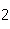c. the number of molecules of nitrogen in 1 mol Nd. the number of molecules of carbon monoxide in 1 mol CO

15.

For which of the following conversions does the value of the conversion factor depend upon the formula of the substance?
 a. volume of gas (STP) to moles b. density of gas (STP) to molar mass c. mass of any substance to moles d. moles of any substance to number of particles

16.

The volume of one mole of a substance is 22.4 L at STP for all ____.
 a. gases b. liquids c. solids d. compounds

17.

What is the formula mass of ethyl alcohol, C2H5OH?
 a. 30.33 amu b. 33.27 amu c. 45.06 amu d. 46.08 amu

18.

How many oxygen atoms are there in 0.500 mol of CO2?
 a. 6.02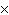1023 b. 3.011023 c. 15.9994 d. 11

19.

How many moles of calcium are in 425 g calcium (Ca)?
 a. 10.6 mol c. 171 mol b. 70.5 mol d. 255 mol

20.

How many molecules are there in 5.0 g of methyl alcohol, CH3OH?
 a. 9.41022 b. 3.01024 c. 3.61024 d. 3.81024

21.

Calculate the number of molecules in 4.0 mol H2O.
 a. 0.60 x 1023 molecules c. 2.4 x 10–23 molecules b. 2.4 x 1024 molecules d. 2.4 x 1023 molecules

22.

What is true about the molar mass of chlorine gas?
 a. The molar mass is 35.5 g. b. The molar mass is 71.0 g. c. The molar mass is equal to the mass of one mole of chlorine atoms. d. none of the above

23.

Given 1.00 mole of each of the following gases at STP, which gas would have the greatest volume?
 a. He b. Oc. SO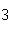d. All are equal.

24.

What is the percent composition of carbon, in heptane, C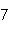H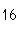?
 a. 12% b. 19% c. 68% d. 84%

25.

The percentage composition of sulfur in SO2 is about 50%. What is the percentage of oxygen in this compound?
 a. 25% b. 50% c. 75% d. 90%

26.

The lowest whole-number ratio of the elements in a compound is called the ____.
 a. empirical formula c. binary formula b. molecular formula d. representative formula

27.

A molecular compound has the empirical formula XY3. Which of the following is a possible molecular formula?
 a. X2Y3 b. XY4 c. X2Y5 d. X2Y6

28.

Which of the following is NOT an empirical formula?
 a. BeCrOc. CNH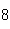b. CHO d. SbS29.

What is the empirical formula for a compound that is 43.6% phosphorus and 56.4% oxygen?
 a. P3O7 b. PO3 c. P2O3 d. P2O5

30.

A compound contains 64 g of O and 8 g of H. What is the empirical formula for this compound?
 a. H2O b. H2O2 c. HO2 d. HO

31.

A compound's empirical formula is N2O5. If the formula mass is 108 amu, what is the molecular formula?
 a. N4O10 b. N2O5 c. NO3 d. N2O4

32.

A compound's empirical formula is CH. If the formula mass is 52 amu, what is the molecular formula?
 a. C4H4 b. C2H2 c. C2H4 d. C4H

33.

What is the empirical formula of a compound that is 40% sulfur and 60% oxygen by weight?
 a. SO b. SOc. SOd. S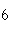O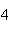34.

Which of the following does not increase the rate of dissolving a solid in water?
 a. raising the temperature of the water b. stirring the solution c. using larger pieces of solid d. crushing the solid

35.

In a concentrated solution there is ____.
 a. no solvent c. a small amount of solute b. a large amount of solute d. no solute

36.

Two liquids that can be mixed together but separate shortly after are:
 a. immiscible b. insoluble c. miscible d. soluble

37.

What is the molarity of a solution that contains 0.202 mol KCl in 7.98 L solution?
 a. 0.0132 M b. 0.0253 M c. 0.459 M d. 1.363 M

38.

What is the molarity of a solution that contains 125 g NaCl in 4.00 L solution? (molar mass of NaCl = 58.44 g/mol)
 a. 0.535 M b. 2.14 M c. 8.56 M d. 31.3 M

39.

What is the molarity of a solution that contains 6 moles of solute in 2 liters of solution?
 a. 6M c. 7M b. 12M d. 3M

40.

Which of the following operations yields the number of moles of solute?
 a. molaritymoles of solution c. molaritymass of solution b. molarityliters of solution d. moles of solution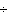volume of solution

41.

What mass of NaSOis needed to make 2.5 L of 2.0M solution? (Na = 23 g; S = 32 g; O = 16 g)
 a. 178 g b. 284 g c. 356 g d. 710 g

42.

Which of the following is NOT true about empirical and molecular formulas?
 a. The molecular formula of a compound can be the same as its empirical formula. b. The molecular formula of a compound can be some whole-number multiple of its empirical formula. c. Several compounds can have the same empirical formula, but have different molecular formulas. d. The empirical formula of a compound can be triple its molecular formula.